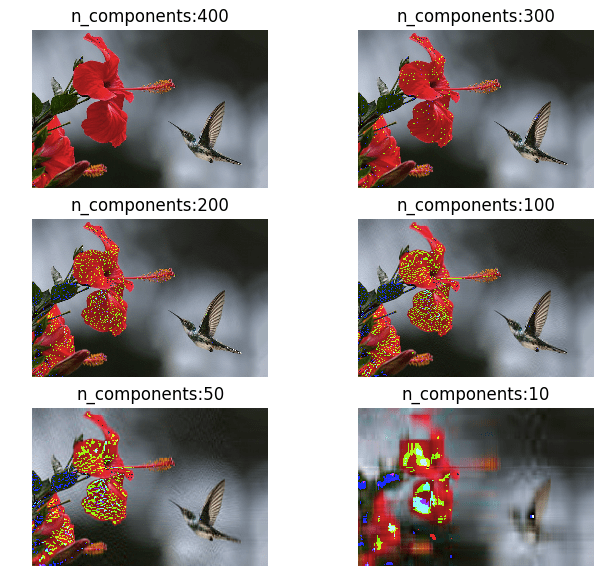# Artificial Intelligence

## All Tesseract OCR options

This is for my reference and this might come in handy for others too. All Tesseract options \$ tesseract –help-extra CLI Examples Command Example Notes tesseract sample_images/image2.jpg stdout To print the output to standard output tesseract sample_images/image2.jpg sample_images/output By default the output will be named outbase.txt. tesseract sample_images/image2.jpg sample_images/output -l eng -l is for language. …## Segmenting lines in handwritten documents using A* Path planning algorithm

In this article, I will explain a widely used method for segmenting handwritten documents into individual lines. Below is a sample output from my algorithm. The below flowchart outlines the different steps involved in the segmentation process. The explained method will only work with non-skewed documents. To de-skew the document, you can refer to my …## Deskewing scanned documents using horizontal projections

An important pre-processing step in any OCR tool or algorithm is to deskew the scanned document first. Take a look at the below sample scanned image, its tilted by a small angle. In this article I will explain a method to deskew these types of documents using their horizontal projections. The final outcome of deskewing …## Mathematics of Principal component analysis

Principal component analysis is a method used to reduce the number of dimensions in a dataset without losing much information. It’s used in many fields such as face recognition and image compression, and is a common technique for finding patterns in data and also in the visualization of higher dimensional data. PCA is all about …

## K-Means on Iris Dataset

Read my previous post to understand how K-Means algorithm works. In this post I will try to run the K-Means on Iris dataset to classify our 3 classes of flowers, Iris setosa, Iris versicolor, Iris virginica (our classess) using the flowers sepal-length, sepal-width, petal-length and petal-width (our features) Getting data: import numpy as np import matplotlib.pyplot …

## Mathematics behind K-Mean Clustering algorithm

K-Means is one of the simplest unsupervised clustering algorithm which is used to cluster our data into K number of clusters. The algorithm iteratively assigns the data points to one of the K clusters based on how near the point is to the cluster centroid. The result of K-Means algorithm is: K number of cluster …

## Understanding Support vector Machines using Python

Support Vector machines (SVM) can be used for both classification as well as regression tasks but they are mostly used in classification applications. Some of the real world applications include Face detection, Handwriting detection, Document categorisation, SPAM Filtering, image classification and protein remote homology detection. For many researchers, SVM is the first best choice for …

## Maths behind Polynomial regression

Polynomial regression is a process of finding a polynomial function that takes the form f( x ) = c0 + c1 x + c2 x2 ⋯ cn xn where n is the degree of the polynomial and c is a set of coefficients. Through polynomial regression we try to find an nth degree polynomial function which is the closest approximation of our data points. Below is a sample random dataset which has been regressed …

## Evaluating the fitness of a modal with a cost function

Previously we derived a simple linear regression modal for our Pizza price dataset. We built a modal that predicted a price of \$13.68 for a 12 inch pizza. When the same modal is used to predict the price of an 8 inch pizza, we get \$9.78 which is around \$0.78 more than the known price of \$9. …

## Math behind Linear Regression with Python code

Simple linear regression is a statistical method you can use to study relationships between two continuous (quantitative) variables: independent variable (x) – also referred to as predictor or explanatory variable dependant variable (y) – also referred to as response or outcome The goal of any regression model is to predict the value of y (dependant variable) based on the …